# Сборник текстов на казахском, русском, английском языках для формирования навыков по видам речевой деятельности обучающихся уровней среднего образования

 бет 31/65 Дата 05.11.2016 өлшемі 17,36 Mb.

## Allotrope of Carbon

An element, in different forms, having different physical properties but similar chemical properties is known as allotropy. Carbon shows allotropy. Such different forms are called 'allotrope' of an element or allotropic forms. There are three well known allotropic forms of carbon and they are amorphous carbon, diamond and graphite. The fourth allotropic form of carbon is buckminsterfullerenes which is basically an artificial form of carbon and is made up of 60 C atoms.

A few examples of pure carbons are as follows:

Coal, Coke, Charcoal (or wood charcoal), Animal Charcoal (or bone black), Lamp black, Carbon black,

Gas carbon and Petroleum coke

Diamonds and graphite are two crystalline allotropes of carbon. Diamond and graphite both are covalent crystals. But, they differ considerably in their properties.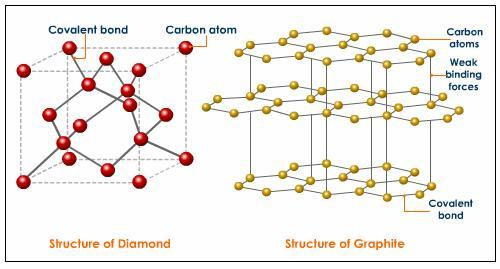Comparison of the Properties of Diamond and Graphite

 Diamond Graphite It occurs naturally in free state. It occurs naturally and is manufactured artificially. It is the hardest natural substance known. It is soft and greasy to touch. It has high relative density (about 3.5). Its relative density is 2.3. It is transparent and has high refractive index (2.45). It is black in color and opaque. It is non-conductor of heat and electricity. Graphite is a good conductor of heat and electricity. It burns in air at 900°C to give CO2. It burns in air at 700-800°C to give CO2. It occurs as octahedral crystals. It occurs as hexagonal crystals. It is insoluble in all solvents. It is insoluble in all ordinary solvents

These differences in the properties of diamond and graphite are due to the difference in their structures. In diamond, each C atom is linked to its neighbors by four single covalent bonds. This leads to a three-dimensional network of covalent bonds. In graphite, the carbon atoms are arranged in flat parallel layers as regular hexagons.

Each carbon in these layers is bonded to three others by covalent bonds. Graphite thus acquires some double bond character. Each layer is bonded to adjacent layers by weak van der Waals forces. This allows each layer to slide over the other easily. Due to this type of structure graphite is soft and slippery, and can act as a lubricant.
Biochemistry
Biochemistry is the branch of science that explores the chemical processes within and related to living organisms. It is a laboratory based science that brings together biology and chemistry. By using chemical knowledge and techniques, biochemists can understand and solve biological problems.Biochemistry focuses on processes happening at a molecular level. It focuses on what’s happening inside our cells, studying components like proteins, lipids and organelles. It also looks at how cells communicate with each other, for example during growth or fighting illness. Biochemists need to understand how the structure of a molecule relates to its function, allowing them to predict how molecules will interact.

Biochemistry covers a range of scientific disciplines, including genetics, microbiology, forensics, plant science and medicine. Because of its breadth, biochemistry is very important and advances in this field of science over the past 100 years have been staggering. It’s a very exciting time to be part of this fascinating area of study.

What do biochemists do?
Provide new ideas and experiments to understand how life works

Support our understanding of health and disease

Contribute innovative information to the technology revolution

Work alongside chemists, physicists, healthcare professionals, policy makers, engineers and many more professionals

Biochemistry, study of the chemical substances and processes that occur in plants, animals, and microorganisms and of the changes they undergo during development and life. It deals with the chemistry of life, and as such it draws on the techniques of analytical, organic, and physical chemistry, as well as those of physiologists concerned with the molecular basis of vital processes. All chemical changes within the organism—either the degradation of substances, generally to gain necessary energy, or the buildup of complex molecules necessary for life processes—are collectively termed metabolism. These chemical changes depend on the action of organic catalysts known as enzymes, and enzymes, in turn, depend for their existence on the genetic apparatus of the cell. It is not surprising, therefore, that biochemistry enters into the investigation of chemical changes in disease, drug action, and other aspects of medicine, as well as in nutrition, genetics, and agriculture.

Atoms
We have now seen that different materials have different properties. Some materials are metals and some are non-metals; some are electrical or thermal conductors, while others are not. Depending on the properties of these materials, they can be used in lots of useful applications. But what is it exactly that makes up these materials? In other words, if we were to break down a material into the parts that make it up, what would we find? And how is it that a material’s microscopic structure is able to give it all these different properties? The answer lies in the smallest building block of matter: the atom. It is the type of atoms, and the way in which they are arranged in a material, that affects the properties of that substance. It is not often that substances are found in atomic form. Normally, atoms are bonded to other atoms to form compounds or molecules. It is only in the noble gases (e.g. helium, neon and argon) that atoms are found individually and are not bonded to other atoms. We will look at the reasons for this in a later chapter.
The Atom
We have now looked at many examples of the types of matter and materials that exist around us, and we have investigated some of the ways that materials are classified. But what is it that makes up these materials? And what makes one material different from another? In order to understand this, we need to take a closer look at the building block of matter, the atom. Atoms are the basis of all the structures and organisms in the universe. The planets, the sun, grass and trees, the air we breathe, and people are all made up of different combinations of atoms.

Models of the Atom

It is important to realise that a lot of what we know about the structure of atoms has been

developed over a long period of time. This is often how scientific knowledge develops, with one person building on the ideas of someone else. We are going to look at how our modern understanding of the atom has evolved over time.

The idea of atoms was invented by two Greek philosophers, Democritus and Leucippus in the fifth century BC. The Greek word __oμo_ (atom) means indivisible because they believed that atoms could not be broken into smaller pieces.

Nowadays, we know that atoms are made up of a positively charged nucleus in the centre

surrounded by negatively charged electrons. However, in the past, before the structure of the atom was properly understood, scientists came up with lots of different models or pictures to describe what atoms look like.

How big is an atom?

It is difficult sometimes to imagine the size of an atom, or its mass, because we cannot see them, and also because we are not used to working with such small measurements. How heavy is an atom?

It is possible to determine the mass of a single atom in kilograms. But to do this, you would need very modern mass spectrometers, and the values you would get would be very clumsy and difficult to use. The mass of a carbon atom, for example, is about 1.99 x 10−26kg, while the mass of an atom of hydrogen is about 1.67 x 10−27kg. Looking at these very small numbers makes it difficult to compare how much bigger the mass of one atom is when compared to another.

Molecules
Definition: Molecule

A molecule is a group of two or more atoms that are attracted to each other by relatively

strong forces or bonds.

Almost everything around us is made up of molecules. Water is made up of molecules, each of which has two hydrogen atoms joined to one oxygen atom. Oxygen is a molecule that is made up of two oxygen atoms that are joined to one another. Even the food that we eat is made up of molecules that contain atoms of elements such as carbon, hydrogen and oxygen that are joined to one another in different ways. All of these are known as small molecules because there are only a few atoms in each molecule. Giant molecules are those where there may be millions of atoms per molecule. Examples of giant molecules are diamonds, which are made up of millions of carbon atoms bonded to each other, and metals, which are made up of millions of metal atoms bonded to each other.

Representing molecules

The structure of a molecule can be shown in many different ways. Sometimes it is easiest to show what a molecule looks like by using different types of diagrams, but at other times, we may decide to simply represent a molecule using its chemical formula or its written name.

Using formulae to show the structure of a molecule

A chemical formula is an abbreviated (shortened) way of describing a molecule, or some

other chemical substance. In chapter 1, we saw how chemical compounds can be repre-

sented using element symbols from the Periodic Table. A chemical formula can also tell

us the number of atoms of each element that are in a molecule, and their ratio in that

molecule.

For example, the chemical formula for a molecule of carbon dioxide is: CO2

The formula above is called the molecular formula of that compound. The formula tells

us that in one molecule of carbon dioxide, there is one atom of carbon and two atoms of

oxygen. The ratio of carbon atoms to oxygen atoms is 1:2.

Definition: Molecular formula

A concise way of expressing information about the atoms that make up a particular chemical compound. The molecular formula gives the exact number of each type of atom in the molecule.

A molecule of glucose has the molecular formula: C6H12O6

In each glucose molecule, there are six carbon atoms, twelve hydrogen atoms and six oxygen atoms. The ratio of carbon: hydrogen: oxygen is 6:12:6. We can simplify this ratio to write 1:2:1, or if we were to use the element symbols, the formula would be written as CH2O. This is called the empirical formula of the molecule.

Definition: Empirical formula

This is a way of expressing the relative number of each type of atom in a chemical compound.

In most cases, the empirical formula does not show the exact number of atoms, but rather

the simplest ratio of the atoms in the compound. The empirical formula is useful when we want to write the formula for a giant molecule. Since giant molecules may consist of millions of atoms, it is impossible to say exactly how many atoms are in each molecule. It makes sense then to represent these molecules using their empirical formula. So, in the case of a metal such as copper, we would simply write Cu, or if we were to represent a molecule of sodium chloride, we would simply write NaCl.

Chemical formulae therefore tell us something about the types of atoms that are in a

molecule and the ratio in which these atoms occur in the molecule, but they don’t give us

any idea of what the molecule actually looks like, in other words its shape. Another useful way of representing molecules is to use diagrams. Another type of formula that can be used to describe a molecule is its structural formula. A structural formula uses a graphical representation to show a molecule’s structure (figure 2.1).

Using diagrams to show the structure of a molecule

Diagrams of molecules are very useful because they give us an idea of the space that is

occupied by the molecule, and they also help us to picture how the atoms are arranged in

the molecule. There are two types of diagrams that are commonly used: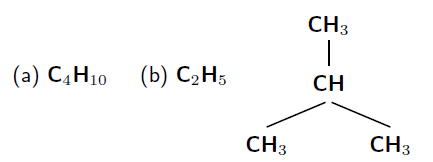Figure 2.1: Diagram showing (a) the molecular, (b) the empirical and (c) the structural formula of isobutane

• Ball and stick models

This is a 3-dimensional molecular model that uses ’balls’ to represent atoms and sticks’ to represent the bonds between them. The centres of the atoms (the balls) are connected by straight lines which represent the bonds between them. A simplified example is shown in figure 2.2.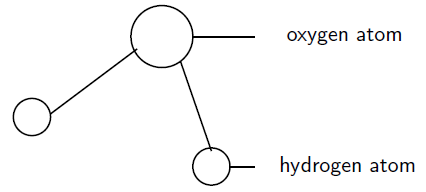Figure 2.2: A ball and stick model of a water molecule

• Space-filling model

This is also a 3-dimensional molecular model. The atoms are represented by multi-

coloured spheres. Space-filling models of water and ammonia are shown in figures

2.3 and 2.4.

Figures 2.3 and 2.4 are some examples of simple molecules that are represented in different ways.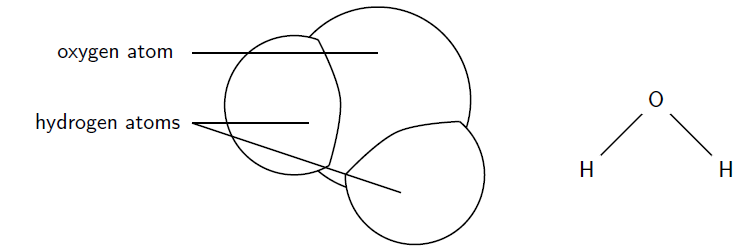Figure 2.3: A space-filling model and structural formula of a water molecule. Each molecule is made up of two hydrogen atoms that are attached to one oxygen atom. This is a simple molecule.

Ions
In the previous section, we focused our attention on the electron configuration of neutral atoms.

In a neutral atom, the number of protons is the same as the number of electrons. But what

happens if an atom gains or loses electrons? Does it mean that the atom will still be part of the same element?

A change in the number of electrons of an atom does not change the type of atom that it is. However, the charge of the atom will change. If electrons are added, then the atom will become more negative. If electrons are taken away, then the atom will become more positive. The atom that is formed in either of these cases is called an ion. Put simply, an ion is a charged atom.

Definition: Ion

An ion is a charged atom. A positively charged ion is called a cation e.g. Na+, and a

negatively charged ion is called an anion e.g. F−. The charge on an ion depends on the

number of electrons that have been lost or gained.

Look at the following examples. Notice the number of valence electrons in the neutral atom, the number of electrons that are lost or gained, and the final charge of the ion that is formed.

Lithium

A lithium atoms loses one electrons to form a positive ion (figure 3.11).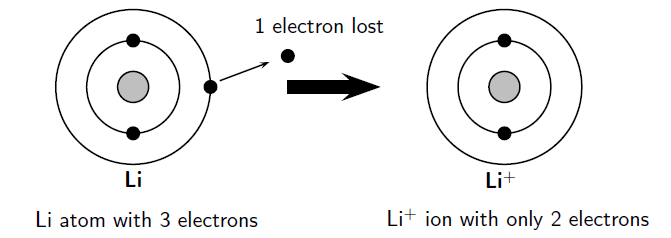The arrangement of electrons in a lithium ion.

In this example, the lithium atom loses an electron to form the cation Li+.

Fluorine

A fluorine atom gains one electron to form a negative ion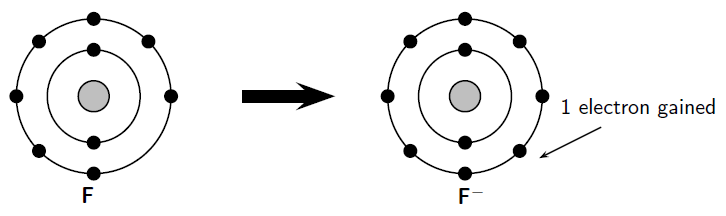The arrangement of electrons in a fluorine ion.

Atomic structure
As a result of the models that we discussed in section 3.1, scientists now have a good idea of what an atom looks like. This knowledge is important because it helps us to understand things like why materials have different properties and why some materials bond with others. Let us now take a closer look at the microscopic structure of the atom.

So far, we have discussed that atoms are made up of a positively charged nucleus surrounded by one or more negatively charged electrons. These electrons orbit the nucleus.

The Electron

The electron is a very light particle. It has a mass of 9.11 x 10−31 kg. Scientists believe that the electron can be treated as a point particle or elementary particle meaning that it can’t be broken down into anything smaller. The electron also carries one unit of negative electric charge which is the same as 1.6 x 10−19 C (Coulombs).

The Nucleus

Unlike the electron, the nucleus can be broken up into smaller building blocks called protons and neutrons. Together, the protons and neutrons are called nucleons.

The Proton

Each proton carries one unit of positive electric charge. Since we know that atoms are electrically neutral, i.e. do not carry any extra charge, then the number of protons in an atom has to be the same as the number of electrons to balance out the positive and negative charge to zero. The total positive charge of a nucleus is equal to the number of protons in the nucleus. The proton is much heavier than the electron (10 000 times heavier!) and has a mass of 1.6726 x 10−27 kg.

When we talk about the atomic mass of an atom, we are mostly referring to the combined mass of the protons and neutrons, i.e. the nucleons.

The Neutron

The neutron is electrically neutral i.e. it carries no charge at all. Like the proton, it is much heavier than the electron and its mass is 1.6749 x 10−27 kg (slightly heavier than the proton).
Rutherford predicted (in 1920) that another kind of particle must be present in the nucleus along with the proton. He predicted this because if there were only positively charged protons in the nucleus, then it should break into bits because of the repulsive forces between the like-charged protons! Also, if protons were the only particles in the nucleus, then a helium nucleus (atomic number 2) would have two protons and therefore only twice the mass of hydrogen. However, it is actually four times heavier than hydrogen. This suggested that there must be something else inside the nucleus as well as the protons. To make sure that the atom stays electrically neutral, this particle would have to be neutral itself. In 1932 James Chadwick discovered the neutron and measured its mass.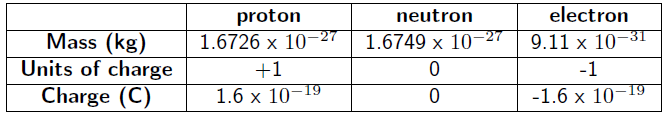1.3 Unlike the electron which is thought to be a point particle and unable to be

broken up into smaller pieces, the proton and neutron can be divided. Protons

and neutrons are built up of smaller particles called quarks. The proton and

neutron are made up of 3 quarks each.

The chemical properties of an element are determined by the charge of its nucleus, i.e. by the number of protons. This number is called the atomic number and is denoted by the letter Z.

Definition: Atomic number (Z)

The number of protons in an atom.

The mass of an atom depends on how many nucleons its nucleus contains. The number of

nucleons, i.e. the total number of protons plus neutrons, is called the atomic mass number

and is denoted by the letter A.

Definition: Atomic mass number (A)

The number of protons and neutrons in the nucleus of an atom.

Standard notation shows the chemical symbol, the atomic mass number and the atomic number of an element as follows: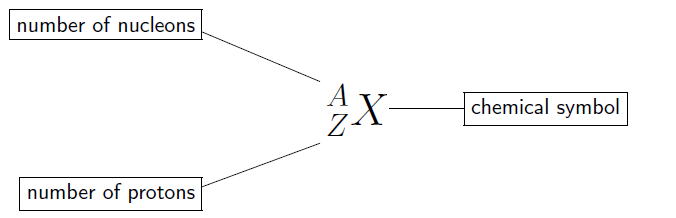A

For example, the iron nucleus which has 26 protons and 30 neutrons, is denoted as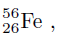where the total nuclear charge is Z = 26 and the mass number A = 56. The number of neutrons is simply the difference N = A − Z.

Important:

Don’t confuse the notation we have used above, with the way this information appears

on the Periodic Table. On the Periodic Table, the atomic number usually appears in the

top lefthand corner of the block or immediately above the element’s symbol. The number

below the element’s symbol is its relative atomic mass. This is not exactly the same as

the atomic mass number. This will be explained in section 3.5. The example of iron is used again below.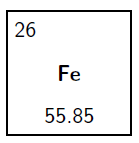You will notice in the example of iron that the atomic mass number is more or less the same as its atomic mass. Generally, an atom that contains n protons and neutrons (i.e. Z = n), will have a mass approximately equal to n u. The reason is that a C-12 atom has 6 protons, 6 neutrons and 6 electrons, with the protons and neutrons having about the same mass and the electron mass being negligible in comparison.

The division of lelectrons from an atom
A large local charge separation usually results when a shared electron pair is donated unilaterally. The three Kekulé formulas shown here illustrate this condition.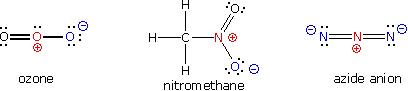In the formula for ozone the central oxygen atom has three bonds and a full positive charge while the right hand oxygen has a single bond and is negatively charged. The overall charge of the ozone molecule is therefore zero. Similarly, nitromethane has a positive-charged nitrogen and a negative-charged oxygen, the total molecular charge again being zero. Finally, azide anion has two negative-charged nitrogens and one positive-charged nitrogen, the total charge being minus one.

In general, for covalently bonded atoms having valence shell electron octets, if the number of covalent bonds to an atom is greater than its normal valence it will carry a positive charge. If the number of covalent bonds to an atom is less than its normal valence it will carry a negative charge. The formal charge on an atom may also be calculated by the following formula: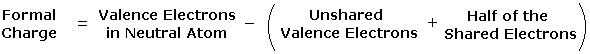Достарыңызбен бөлісу: